# Broken Sticks, Triangles, and Probability I

This week we look at questions about how likely it is that you can make a triangle out of three random pieces of a stick. As always in probability, the first issue comes in deciding how the process is to be done (that is, what does it mean to break a stick randomly?); we’ll also see multiple methods for solving the same problem, which is always interesting. To keep this post from being too long, I’ll be showing two methods for solving one interpretation of the problem here, and then show a third method next week, along with a related method for a second interpretation.

## Introduction: Two interpretations

Our first question is from 1995, months after Ask Dr. Math started:

Break a dowel to form a triangle

Hi, I have a problem:

A wooden dowel is randomly broken in 2 places.  What is the probability that the 3 resulting fragments can be used to form the sides of a triangle?

Thanks.

Sometimes you can make a triangle, sometimes you can’t: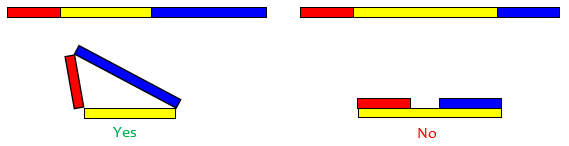How likely is it that you can, if you make the breaks randomly? Try making a guess before reading ahead; do you think it will be rare or common?

I'd say it makes a difference how it's broken and what you mean by random.  For instance, is the stick broken once and then one of two pieces is selected at random to be broken again ("pick one and break again")?  Or do you pick two spots on the dowel at random where the breaks will be made (two-at-once)?

Let's look at the second situation because the first seems similar but a little harder to calculate.


We’ll be spending most of our time on the second, “two-at-once” interpretation; next week we’ll see a variation of the first, which seems most natural to me: “break, then break the longer piece again”.

### Choosing both locations, then cutting

In reality, if you broke a stick “randomly” (that is, according to informal ideas of randomness), you would probably have a bias against leaving a tiny piece, and would tend toward breaking into approximately equal parts – especially if you were literally breaking them, which requires some leverage. We’re talking instead about actual random choices, which might be accomplished by picking a random real number between zero and one, marking the stick at that location, and repeating for the second mark before actually breaking or cutting.

But before starting the actual work, it’s interesting to make some comparisons:

Two-at-once is like "pick one and break again" except that with the latter we have a fifty percent chance of choosing the bigger piece for the second break; whereas for two-at-once, since the location of the second break is made with reference to the whole stick, you have a greater than fifty percent chance of making the next break in the bigger piece since the bigger piece is more than fifty percent of the whole stick.  So the probability of "two-at-once" resulting in a triangle should be better than that of "pick one and break again."

By similar reasoning, the probability in “break the longer”, as we’ll see, turns out to be still higher.

Now, what does it take for three sticks to be able to make a triangle? Will any three sticks do?  Once you express the minimum conditions the broken pieces must satisfy in relation to each other if they are to make a triangle, then you can make 3 statements, in this case, 3 inequalities to solve. Try to figure this out before reading the "spoiler" below.  When I set up my equations, I let x be the distance from the left side of the dowel to the first break. Let y be the distance from the left side to the second break. Now we have two sets of inequalities. When y is to the right of x, we have one set. When y is to the left of x, we have another.  But we can see that these two situations are equally likely, in fact the probability in each case should be the same, so once we have calculated one the total will be easy to figure.

The condition for making a triangle from three lengths a, b, and c, is the triangle inequality, which can be applied to each of the three sides: $$a+b>c\\b+c>a\\c+a>b$$ This amounts to the fact that a straight line is the shortest path between two points.

*********************
"y to the right of x"
*********************

("Semi-Spoiler")

The lengths of the pieces are x, 1-y, y-x. So we can set up three inequalities since any two sides added together have to be longer than the third.

What happens if you set up these inequalities and solve them?  I'll leave it to you to write out the inequalities.

Our inequalities are x+(1-y)>y-x\$$1-y)+(y-x)>x\\(y-x)+x>1-y which simplify to y-x<\frac{1}{2}\\x<\frac{1}{2}\\y>\frac{1}{2} For the case with y to the left, the corresponding inequalities will be x-y<\frac{1}{2}\\y<\frac{1}{2}\\x>\frac{1}{2} ### Finding the probability as an area When doing problems such as this I like to make a 1 by 1 square, the area of which represents the total probability (1). Let's say the length of the unbroken stick is one unit. The horizontal side of our square could represent the first break which is anywhere from 0 to 1. The vertical side of our square will be the second break, y. If you graph the inequalities you get a triangular area occupying the lower right hand half of the upper left quadrant. The area of that triangle is equal to the probability of "y to the right of x". 1| /| | / | | / | 1/2| /_ _ _ | | | |_ _ _ _ _ _ _ _ _ 0 1/2 1 Don't forget about "y to the left of x". The lines on this graph are \(x=\frac{1}{2}$$, $$y=\frac{1}{2}$$, $$y=x+\frac{1}{2}$$, corresponding to our three inequalities.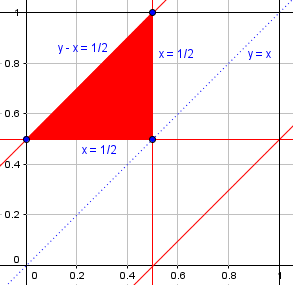We’ll be seeing this graph, in its complete form, soon.

Once you have completed this version of the problem you might want to go back and see if you can set up the inequalities for "pick one and break again".  The idea is the same but the math can be harder.

We’ll get to that at the end of next week’s post. First, we’ll finish this solution for the problem we’ve been working on, and two more.

## Solution A: In two dimensions

The next question, from 1997, assumes our two-cut interpretation:

Probability of a Triangle

If I cut a 12-inch ruler with two arbitrary cuts, what is the probability that from the three pieces created I will be able to create a triangle?

I came up with 25 percent probability.  Is that correct?

Chez

This time the stick length is given as 12 inches rather than 1 foot, but that has no effect on the problem. And the answer is correct, though we don’t know Chez’ method.

Doctor Anthony answered, offering the same method of solution we’ve started, but finishing it:

These problems are best done by considering two random variables x and y on the usual rectangular axes, with both x and y uniformly distributed between 0 and 12. The probability space is a square of side 12, and we need to consider regions of the square that lead to three lengths which can form a triangle.

The probability space is a representation of all possibilities (x, y), and since we are supposing that x and y are independently chosen with uniform probability distribution, the probability of any event (region in this space) is the area of the region divided by the area of the entire square: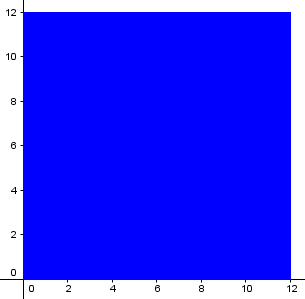Suppose that the breaks are made at points distance x and y from the one end. Then if y > x (we can consider the alternative later) the three lengths will be x, y-x and 12-y. For a triangle any two of these must be greater than the third, so

x + y-x > 12-y         y-x + 12-y > x           x + 12-y > y-x

y > 6                  x < 6                    y < x+6

If you plot these three boundaries you get a triangle with vertices at

(6,6) (0,6) (6,12)  and area equal 1/8 of the square.

All of this is the equivalent in inches of what we already did in feet: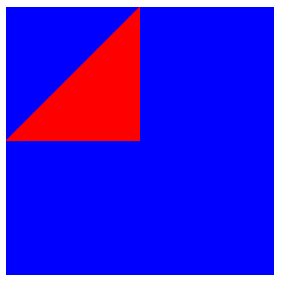This assumed y > x.  If instead x > y then we get a second region symmetrically placed below the diagonal y=x of the square and again having an area 1/8 the area of the square. So the total area of the square giving values of x and y which allow for a triangle to be formed is 1/8 + 1/8 = 1/4 .

So the probability that a triangle can be formed is 1/4.

Here is the square, with the event “triangle is possible” in red, showing a point P(x, y) representing a possible triangle: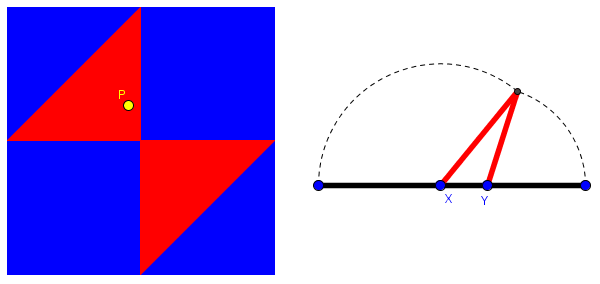Here is a point representing an impossible triangle: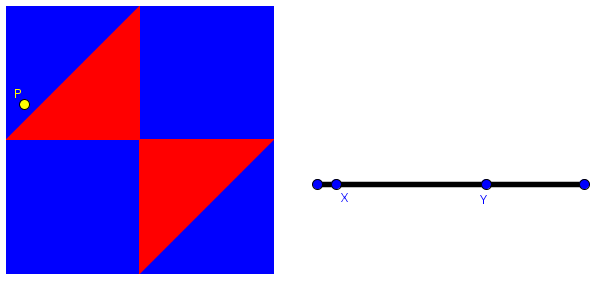(These pictures come from a GeoGebra sketch I made that plots the point P and makes the triangle for any positions of points X and Y.)

## Solution B: In three dimensions

Here is a 2001 version of the same question:

Probability of Forming a Triangle

I don't know where to begin.

A rope one unit long is cut in two places. What is the probability that the three resulting pieces can be arranged to form a triangle?

Doctor Pat answered, with a very different solution from Dan Asimov:

Mike,

Here is one way, I got this as an answer from the nephew of the late Isaac Asimov, the Science writer, when I posed this problem on the Internet several years ago. Dan is a very good mathematician on his own, as you can see from his approach to the problem...

If we let T denote the planar triangle in 3-space whose vertices are (1,0,0), (0,1,0), and (0,0,1), then choosing a point p at random on T (i.e. with the probability of p lying in a subset S of T being proportional to the area of S, or more precisely Prob(p is in S) = area(S) / area(T)), will simulate the random cutting of the unit segment described above, with resulting pieces of lengths x, y, and z respectively.

This time, rather than taking x and y as locations on the stick/rope, we take x, y, and z as the lengths of the three pieces, so that $$x+y+z=1$$. That is the equation of the plane containing our triangle T, which therefore represents all possible sets of (positive) lengths resulting from cutting. It is reasonable (though not proved here) that probability is proportional to area.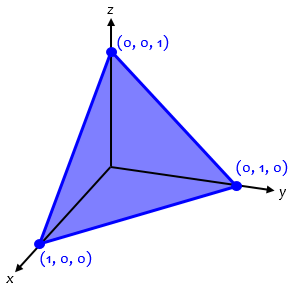The condition that x, y, and z "will form a triangle" is equivalent to the three conditions:  x + y > z, x + z > y, and y + z > x.  The subset of T where (x,y,z) satisfy these three conditions turns out to correspond to the little triangle whose vertices are the midpoints of the edges of T. Therefore Prob(the 3 pieces will form a triangle) is one-fourth.

Here is the midpoint triangle: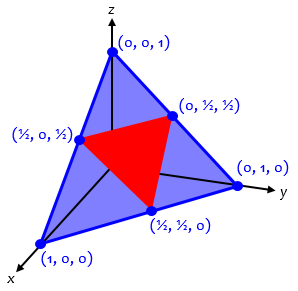To which I added...

From the three conditions above, you can also deduce that the conditions  x + y > z, x + z > y, and y + z > x with the additional knowledge that x + y + z = 1 reduces to Max(x,y,z) < .5,  which offers another easy approach...

This just saves us from having to separately plot the planes $$x+y=z$$, $$x+z=y$$, and $$y+z=x$$, and seeing that they intersect T in the edges of the midpoint triangle. But also, the fact noted here, that x, y, and z must all be less than $$\frac{1}{2}$$, will be the key to the third solution we’ll see next week.

After that, we’ll see the same technique applied to another interpretation of the problem (which is why I want to put them together.)

### 1 thought on “Broken Sticks, Triangles, and Probability I”

This site uses Akismet to reduce spam. Learn how your comment data is processed.